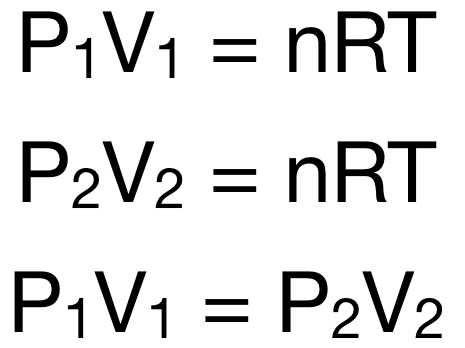# Problem: A 12 Liter tank contains helium gas pressurized to 160 atm.How many 3-liter balloons could the 12 Liter helium tank fill?(Keep in mind that an "exhausted" helium tank is not empty. In other words, once the gas inside the tank reaches atmospheric pressure, it will no longer be able to fill balloons.)

###### FREE Expert Solution
88% (448 ratings)
###### FREE Expert Solution

Recall: The ideal gas law is given by the equation: PV = nRT

At constant temperature and moles of gas:The equation P1V1 = P2V2 is Boyle's Law, which states that the pressure of a certain amount of gas at a certain temperature is inversely proportional to its volume, i.e. the pressure decreases as volume increases.

88% (448 ratings)###### Problem Details

A 12 Liter tank contains helium gas pressurized to 160 atm.

How many 3-liter balloons could the 12 Liter helium tank fill?

(Keep in mind that an "exhausted" helium tank is not empty. In other words, once the gas inside the tank reaches atmospheric pressure, it will no longer be able to fill balloons.)

What scientific concept do you need to know in order to solve this problem?

Our tutors have indicated that to solve this problem you will need to apply the Chemistry Gas Laws concept. If you need more Chemistry Gas Laws practice, you can also practice Chemistry Gas Laws practice problems.

What is the difficulty of this problem?

Our tutors rated the difficulty ofA 12 Liter tank contains helium gas pressurized to 160 atm.H...as high difficulty.

How long does this problem take to solve?

Our expert Chemistry tutor, Dasha took 4 minutes and 13 seconds to solve this problem. You can follow their steps in the video explanation above.

What professor is this problem relevant for?

Based on our data, we think this problem is relevant for Professor Randles' class at UCF.